# Quadratic formula to find n^(th) term of a sequence very easy method - consistent difference between differences

## Method 3

This method is very difficult to remember but very quick to work out.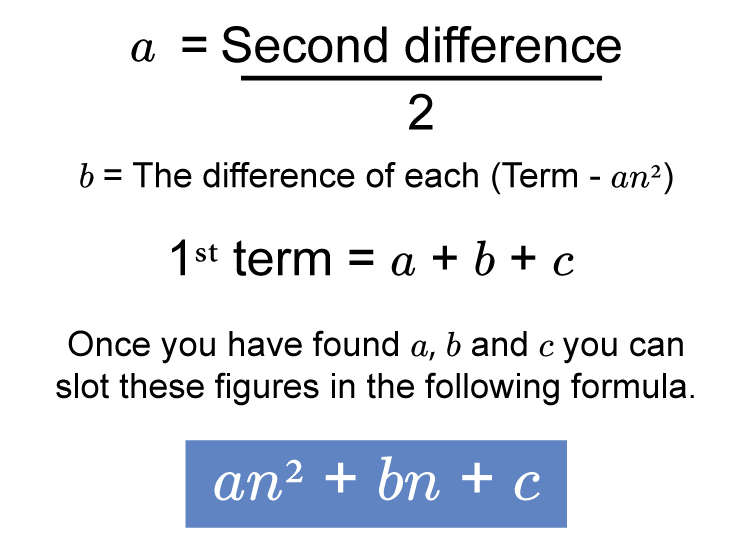Example 1

We know the following sequence is a quadratic sequence but what is the formula for the n^(th) term?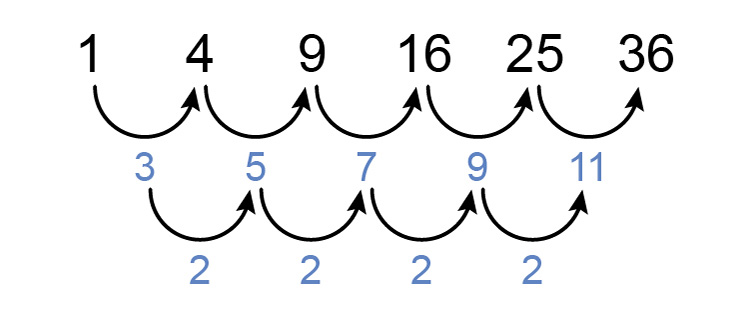a=(se\cond\ \ di\fference)/2=2/2=1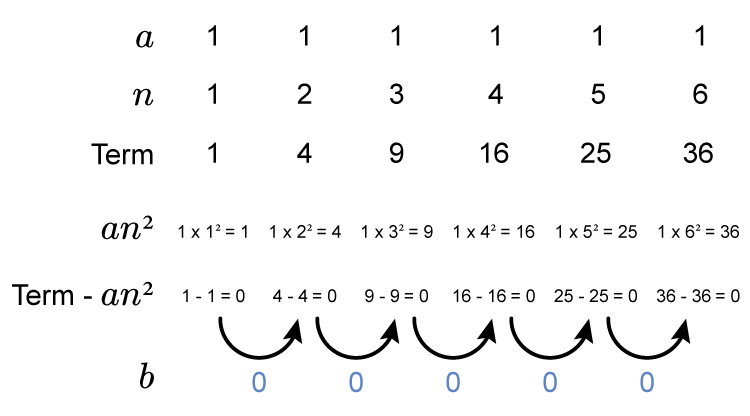Therefore b=0

and because 1^(st) term =a+b+c

1=1+0+c

1-1=0+c

c=0

Summary  a=1   b=0   c=0

therefore the formula for the n^(th) term in this sequence is

an^2+bn+c

or 1n^2+0n+0

which is n^2

Now we need to check the formula is correct.

Try different values of n in the formula n^2

If  n=1       term  =1^2=1

If  n=2       term  =2^2=4

If  n=3       term  =3^2=9

If  n=4       term  =4^2=16

This is correct

Answer =n^2

Example 2

We know the following sequence is a quadratic sequence but what is the formula for the n^(th) term?a=(se\cond\ \ di\fference)/2=2/2=1Therefore b=-1

and because 1^(st) term =a+b+c

2=1-1+c

c=2

Summary  a=1   b=-1   c=2

therefore the formula for the n^(th) term in this sequence is

an^2+bn+c

or 1n^2+(-1)n+2

which is n^2-n+2

Now we need to check the formula is correct.

Try different values of n in the formula n^2-n+2

If  n=1       term  =1^2-1+2=1-1+2=2

If  n=2       term  =2^2-2+2=4-2+2=4

If  n=3       term  =3^2-3+2=9-3+2=8

If  n=4       term  =4^2-4+2=16-4+2=14

This is correct

Answer =n^2-n+2

Example 3

We know the following sequence is a quadratic sequence but what is the formula for the n^(th) term?a=(se\cond\ \ di\fference)/2=2/2=1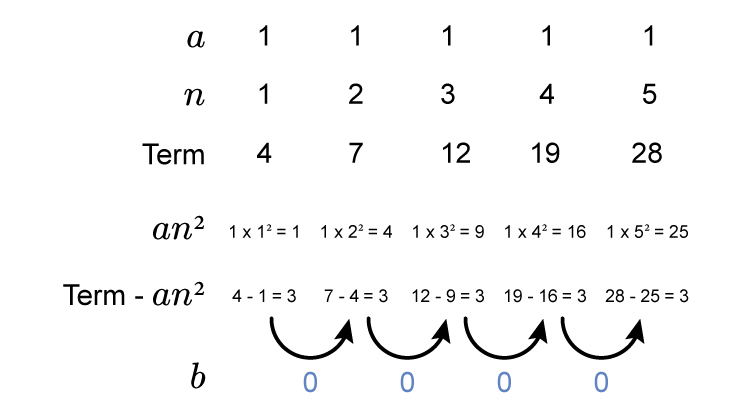Therefore b=0

and because 1^(st) term =a+b+c

4=1+0+c

c=3

Summarry  a=1   b=0   c=3

therefore the formula for the n^(th) term in this sequence is

an^2+bn+c

or 1n^2+0n+3

which is n^2+3

Now we need to check the formula is correct.

Try different values of n in the formula n^2+3

If  n=1       term  =1^2+3=1+3=4

If  n=2       term  =2^2+3=4+3=7

If  n=3       term  =3^2+3=9+3=12

If  n=4       term  =4^2+3=16+3=19

This is correct

Answer =n^2+3

Example 4

We know the following sequence is a quadratic sequence but what is the formula for the n^(th) term?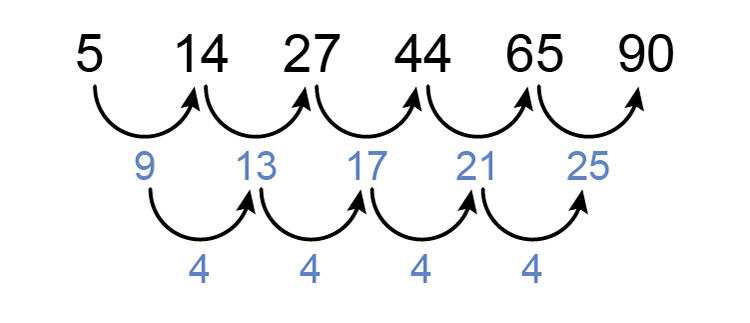a=(se\cond\ \ di\fference)/2=4/2=2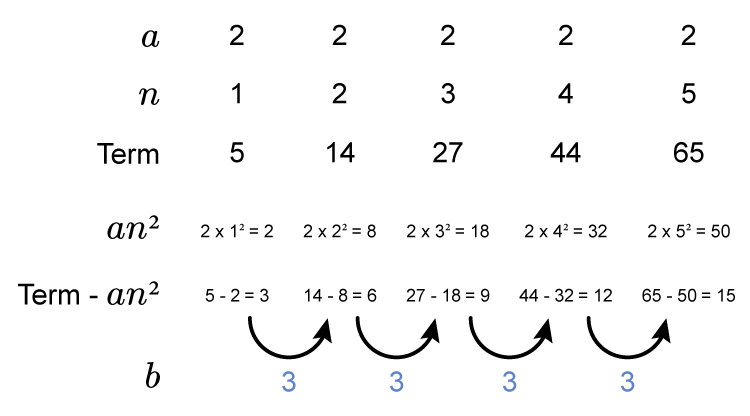Therefore b=3

and because 1^(st) term =a+b+c

5=2+3+c

c=5-2-3

c=0

Summarry  a=2   b=3   c=0

therefore the formula for the n^(th) term in this sequence is

an^2+bn+c

or 2n^2+3n+0

which is 2n^2+3n

Now we need to check the formula is correct.

Try different values of n in the formula 2n^2+3n

If  n=1       term  =2times1^2+3times1=2+3=5

If  n=2       term  =2times2^2+3times2=8+6=14

If  n=3       term  =2times3^2+3times3=18+9=27

If  n=4       term  =2times4^2+3times4=32+12=44

This is correct

Answer =2n^2+3n